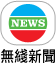1.

2.

3.

4.

5.

6.

A.

B.

C.

D.

E.

F.

G.

H.

I.

J.

K.

L.

M.

N.

O.

P.

Q.

R.

8.

9.

10.

A.

B.

C.

D.

E.

F.

12.

A.

B.

C.

14.

15.

### 披露

16.

A.

B.

C.

D.

E.
「個人資料用作廣告投放及廣告個性化」第10段中所述之人士。
18.

19.

20.

A.

B.

C.

22.

23.

24.

25.

26.

A.

B.

C.

28.

### 一般

29.

30.

31.

32.

33.

34.